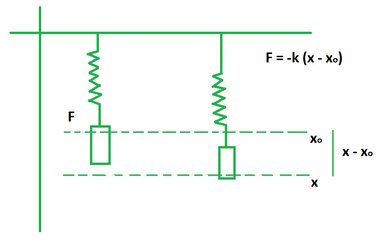# Spring Force Formula

• Last Updated : 01 May, 2022

Spring is a tool which applies an equal and opposite force to a body that compresses or stretches it. This behaviour is shown by the spring because of its negligible inertia. It exhibits harmonic simple motion such that its spring force (or restoring force) is proportional to the displacement. The unit of measurement of the spring force is Newton (N). It is denoted by the symbol F and its dimensional force is given by [M1L1T-2]. Its value is equal to the product of spring constant and displacement.Formula

F = – k(x – xo)

where,

F is the spring force,

xo is the equilibrium position,

x is the spring displacement from the equilibrium position.

The negative symbol indicates that the spring force is a restoring force such that it acts in the opposite direction.

### Sample Problems

Problem 1. Calculate the spring constant for a spring of length 10 cm, it is loaded with 2 kg and gets stretched by 20 cm.

Solution:

We have,

m = 2

x = 20

xo = 10

Calculate the spring force.

F = ma

= 2 (20 – 10)

= 2 (10)

= 20 N

Using the formula for spring constant we get,

k = – F/(x – xo)

= – 20/(20 – 10)

= – 20/10

= -2 N/m

Problem 2. Calculate the spring constant for a spring of length 5 cm, it is loaded with 3 kg and gets stretched by 10 cm.

Solution:

We have,

m = 3

x = 10

xo = 5

Calculate the spring force.

F = ma

= 3 (10 – 5)

= 3 (5)

= 15 N

Using the formula for spring constant we get,

k = – F/(x – xo)

= – 15/(10 – 5)

= – 15/5

= -3 N/m

Problem 3. Calculate the spring constant for a spring of length 7 cm, it is loaded with 2.5 kg and gets stretched by 15 cm.

Solution:

We have,

m = 2.5

x = 15

xo = 7

Calculate the spring force.

F = ma

= 2.5 (15 – 7)

= 2.5 (8)

= 20 N

Using the formula for spring constant we get,

k = – F/(x – xo)

= – 20/(15 – 7)

= – 20/8

= -2.5 N/m

Problem 4. Calculate the spring force for a spring of length 2 cm if it gets stretched by 5 cm. The value of the spring constant is 6 N/m.

Solution:

We have,

k = 6

x = 5

xo = 2

Using the formula for spring force we get,

F = – k(x – xo)

= – 6 (5 – 2)

= -6 (3)

= -18 N

Problem 5. Calculate the spring force for a spring of length 4 cm if it gets stretched by 12 cm. The value of the spring constant is 2 N/m.

Solution:

We have,

k = 2

x = 12

xo = 4

Using the formula for spring force we get,

F = – k(x – xo)

= – 2 (12 – 4)

= -2 (8)

= -16 N

Problem 6. Calculate the spring constant for a spring of length 6 cm if it gets stretched by 18 cm and restores a force of 30 N.

Solution:

We have,

F = 30

x = 18

xo = 6

Using the formula for spring force we get,

k = – F/(x – xo)

= – 30/(18 – 6)

= -30/14

= -2.14 N/m

Problem 7. Calculate the initial length of the spring if it gets stretched by 5 cm and restores a force of 25 N. The value of the spring constant is 5 N/m.

Solution:

We have,

F = 25

x = 5

k = 5

Using the formula for spring force we get,

(x – xo) = -F/k

=> 5 – xo = -25/5

=> xo – 5 = 5

=> xo = 10 cm

My Personal Notes arrow_drop_up
Recommended Articles
Page :